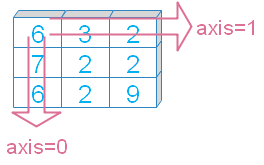# Numpy std()

Numpy

``numpy.std(a, axis=None, dtype=None, out=None, ddof=0, keepdims=)``
Return standard deviation of elements across given axis.
 `a` array, elements to get the std value `axis` Int (optional ), or tuple, default is None, stdvalue among all the elements. If axis given then values across the axis is returned. `out` Optional. If given then output to be stored. Must be of same shape as of the output `keepdims` Bool ( Optional ), output matches to the input array dimension. `ddof` Delta Degrees of Freedom ( default is 0 ) , N - ddof is used where N is the number of elements in computing the standard deviation
We will use these parameters in our examples.

## Sample array

You can use randint() to create an array for our examples. Or can use fixed elements to create the array.
``````import numpy as np
# my_data=np.random.randint(2,high=7,size=(3,3),dtype='int16')
my_data=np.array([[6, 3, 2], [7, 2, 2], [6, 2, 9]])
print(my_data)``````
Output
``````[[6 3 2]
[7 2 2]
[6 2 9]]``````

## Axis

stdimum value of the elements across the axis.``````print("std()       : ", my_data.std())
print("std(axis=0) : ", my_data.std(axis=0))
print("std(axis=1) : ", my_data.std(axis=1))``````
Output
``````std()       :  2.5385910352879693
std(axis=0) :  [0.47140452 0.47140452 3.29983165]
std(axis=1) :  [1.69967317 2.3570226  2.86744176]``````

## out

Alternative output array, must be of same shape as expected output. Let us first check with axis.

## keepdims

If it is set to True ( keepdims=True ) then it will take the dimension of input array.
``````print("std(keepdims=True) : ", my_data.std(keepdims=True))
print("std(keepdims=False) : ", my_data.std(keepdims=False))``````
Output
``````std(keepdims=True) :  [[2.53859104]]
std(keepdims=False) :  2.5385910352879693``````

## ddof

`ddof = 0` ( default) , this is Population Standard Deviation
`ddof = 1` , this is Sample Standard Deviation
Check this code with output.
``````import numpy as np
list1=[12,13,15,11,9,12,13,10,11,12,13,7,8]
my_data=np.array(list1)
print(my_data.std(ddof=0)) # 2.153846153846154
print(my_data.std(ddof=1)) # 2.2417941532712202``````

Subscribe to our YouTube Channel here

## Subscribe

* indicates required
Subscribe to plus2netplus2net.com

Post your comments , suggestion , error , requirements etc here